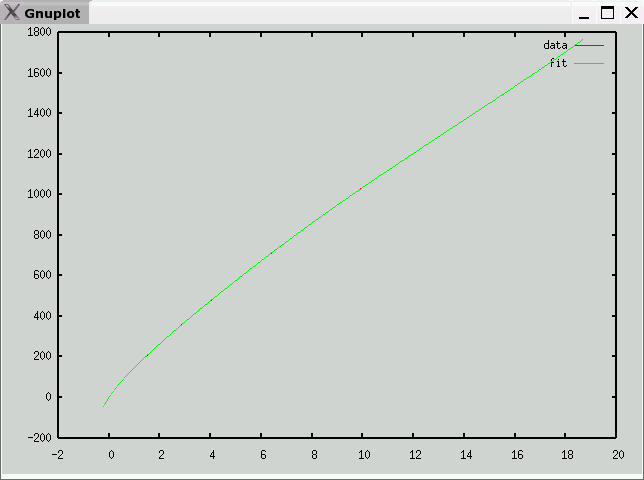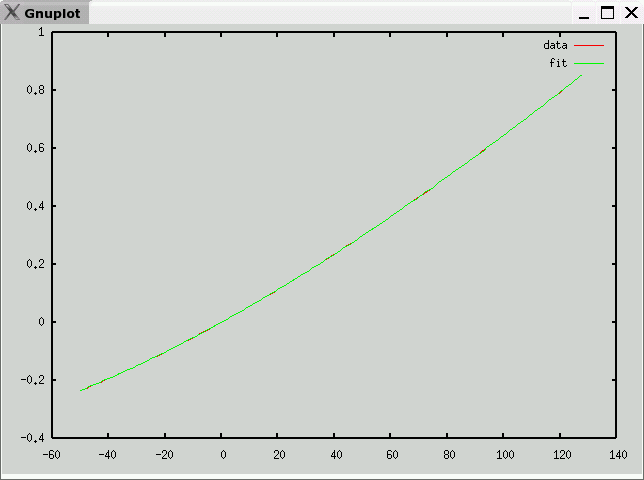# Type S Thermocouple Data and Polynomial

• Description
Pt(10%)-Rh / Pt
• Data source
National Institute of Science and Technology (NIST)
• Raw Data
• Octave format
• Curve fit
• mV -> temperature (C)
e is maximum error (in degrees C)
v1 andf v2 partition the domain into three segments, each with a polynomial fit
c is vector of coefficients: a10, a9, ... a0
Note: Each column of c corresponds to one range (<v1,v1 to v2, >v2)

`e = 0.14051v1 = 7.9810v2 = 11.483c =   -6.4681e-05    8.2531e-04  -1.4220e-05    2.7022e-03   -8.4663e-02   2.1706e-03   -4.8589e-02    3.8894e+00  -1.4849e-01    4.9236e-01   -1.0540e+02   5.9957e+00   -3.0955e+00    1.8665e+03  -1.5825e+02    1.2563e+01   -2.2574e+04   2.8532e+03   -3.3438e+01    1.8886e+05  -3.5590e+04    5.9395e+01   -1.0795e+06   3.0328e+05   -7.6212e+01    4.0349e+06  -1.6898e+06    1.8671e+02   -8.9066e+06   5.5595e+06   -1.8591e-01    8.8186e+06  -8.2014e+06 `• temperature -> mV (range -127C-127C)
e is maximum error (in mV)
c is vector of coefficients: a10, a9, ... a0

`e = 0.00055727c =   -3.6197e-22    1.8568e-19   -3.3883e-17    2.2856e-15    2.4668e-14   -8.7475e-12    2.2200e-10   -1.7509e-08    1.2421e-05    5.4062e-03    2.3179e-05 `Previous page: Type R
Next page: Type T